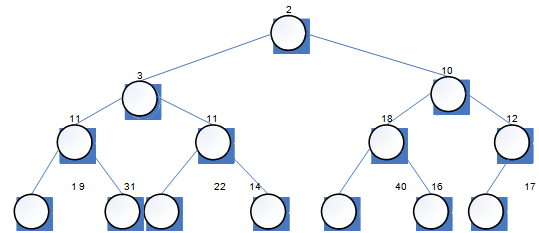## Design a binary tree, Data Structure & Algorithms

Assignment Help:

(a) Suppose that t is a binary tree of integers (that is, an object of type BinTree of Int.) in the state shown in Figure 3.Give the vectors returned by each of the following expressions.

(i) LEVEL_ORDER(t)

(ii) IN_ORDER(t.leftTree( ))

(b) suppose that v is an object of type VectorPlus of mString in the state ["tawny", "little", "barn", "long-eared", "eagle", "scops"]

In answering this part of the question you may, if you wish, use the WorkPad.

(i) Suppose that the strings in the vector v are rearranged into a sorted vector (where the strings in v arc compared using the lexicographic order described on page 32 of Unit 8). G i v e t h e vector that results from this rearrangement

(ii) Suppose that, the function GROW_TREE is applied to the sorted vector which you gave in part (i). Give the binary search tree which is returned by GROW_TREE.

(iii) Suppose that. The string "hawk" is inserted into the binary search tree which you gave in part (ii), using Strategy 3.2 of Unit 8. Give the resulting tree.

(c) The tree in Figure is a heap tree. Use Strategy 3.4 of Unit 8 to remove the root item from that tree. Give the binary tree produced by this strategy.

#### Polynomials, Polynomials like  5x 4    +  2x 3    +  7x 2     +  10x  -  8...

Polynomials like  5x 4    +  2x 3    +  7x 2     +  10x  -  8  can  be  represented by using arrays. Arithmetic operations such as addition & multiplication of polynomials are com

#### Define the term ''complexity of an algorithm, Define the term 'complexity o...

Define the term 'complexity of an algorithm; Complexity of an algorithm is the calculate of analysis of algorithm. Analyzing an algorithm means predicting the resources that th

#### Hashing and hash functions, Q. Describe the term hashing. Explain any two u...

Q. Describe the term hashing. Explain any two usually used hash functions. Explain one method of collision resolution.

#### A binary tree of depth "d" is an almost complete binary tree, A binary tree...

A binary tree of depth "d" is an almost complete binary tree if  A) Every leaf in the tree is either at level "d" or at level "d-1"  B)  For any node "n" in the tree with a

#### What is Oscillating Sort?, For the Oscillating sort to be applied, it is ne...

For the Oscillating sort to be applied, it is necessary for the tapes to be readable in both directions and able to be quickly reversed. The oscillating sort is superior to the po

#### Explain the prim''s minimum spanning tree algorithm, Question 1. Explai...

Question 1. Explain the different types of traversal on binary tree 2. Explain the Prim's minimum spanning tree algorithm 3. Differentiate fixed and variable storage allo

#### Complexity of an algorithm, compare two functions n and 2n for various valu...

compare two functions n and 2n for various values of n. determine when second becomes larger than first

#### Explain all-pair shortest-paths problem, Explain All-pair shortest-paths pr...

Explain All-pair shortest-paths problem Given a weighted linked graph (undirected or directed), the all pairs shortest paths problem asks to find the distances (the lengths of

#### What is diffuse illumination, Diffuse Illumination Diffuse illuminatio...

Diffuse Illumination Diffuse illumination means light that comes from all directions not from one particular source. Think about the light of a grey cloudy day as compared to

#### Determine about the logic gates, Determine about the logic gates Many e...

Determine about the logic gates Many electronic circuits operate using binary logic gates. Logic gates essentially process signals that represent true or false or equivalent i.